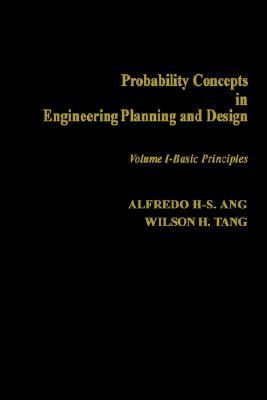118643

9780471032007

# Probability Concepts in Engineering Planning and Design\$64.70
\$3.95 Shipping
List Price
\$122.95
Discount
47% Off
You Save
\$58.25

• Condition: New
• Provider: gridfreed Contact
• Provider Rating:
75%
• Ships From: San Diego, CA
• Shipping: Standard
• Comments: New. In shrink wrap. Looks like an interesting title!30-day money back guarantee
\$1.89
\$3.95 Shipping
List Price
\$122.95
Discount
98% Off
You Save
\$121.06

• Condition: Acceptable
• Provider: Gulf Coast Books Contact
• Provider Rating:
87%
• Ships From: Memphis, TN
• Shipping: Standard30-day money back guarantee

Most renters respond to questions in 48 hours or less.
The response will be emailed to you.
• ISBN-13: 9780471032007
• ISBN: 047103200X
• Publisher: Wiley & Sons, Incorporated, John

### AUTHOR

Ang, Alfredo Huo-Sing, Tang, Wilson H.

### SUMMARY

1. Role of Probability in Engineering 1.1. Introduction 1.2. Uncertainty in Real-World Information 1.2.1. Uncertainty Associated with Randomness 1.2.2. Uncertainty Associated with Imperfect Modeling and Estimation 1.3. Design and Decision-Making Under Uncertainty 1.3.1. Planning and Design of Airport Pavement 1.3.2. Hydrologic Design 1.3.3. Design of Structures and Machines 1.3.4. Geotechnical Design 1.3.5. Construction Planning and Management 1.3.6. Photogrammetric, Geodetic, and Surveying Measurements 1.4. Control and Standards 1.5. Concluding Remarks 2. Basic Probability Concepts 2.1. Events and Probability 2.1.1. Characteristics of Probability Problems 2.1.2. Calculation of Probability 2.2. Elements of Set Theory 2.2.1. Definitions 2.2.2. Combination of Events 2.2.3. Operational Rules 2.3. Mathematics of Probability 2.3.1. Basic Axioms of Probability; Addition Rule 2.3.2. Conditional Probability; Multiplication Rule 2.3.3. Theorem of Total Probability 2.3.4. Bayes' Theorem 2.4. Concluding Remarks Problems 3. Analytical Models of Random Phenomena 3.1. Random Variables 3.1.1. Probability Distribution of a Random Variable 3.1.2. Main Descriptors of a Random Variable 3.2. Useful Probability Distributions 3.2.1. The Normal Distribution 3.2.2. The Logarithmic Normal Distribution 3.2.3. Bernoulli Sequence and the Binomial Distribution 3.2.4. The Geometric Distribution 3.2.5. The Negative Binomial Distribution 3.2.6. The Poisson Process and Poisson Distribution 3.2.7. The Exponential Distribution 3.2.8. The Gamma Distribution 3.2.9. The Hypergeometric Distribution 3.2.10. The Beta Distribution 3.2.11. Other Distributions 3.3. Multiple Random Variables 3.3.1. Joint and Conditional Probability Distributions 3.3.2. Covariance and Correlation 3.3.3. Conditional Mean and Variance 3.4. Concluding Remarks Problems 4. Functions of Random Variables 4.1. Introduction 4.2. Derived Probability Distributions 4.2.1. Function of Single Random Variable 4.2.2. Function of Multiple Random Variables 4.3. Moments of Functions of Random Variables 4.3.1. Introduction 4.3.2. Mean and Variance of a Linear Function 4.3.3. Product of Independent Variates 4.3.4. Mean and Variance of a General Function 4.4. Concluding Remarks Problems 5. Estimating Parameters from Observational Data 5.1. The Role of Statistical Inference in Engineering 5.1.1. Inherent Variability and Estimation Error 5.2. Classical Approach to Estimation of Parameters 5.2.1. Random Sampling and Point Estimation 5.2.2. Interval Estimation of the Mean 5.2.3. Problems of Measurement Theory 5.2.4. Interval Estimation of the Variance 5.2.5. Estimation of Proportion 5.3. Concluding Remarks Problems 6. Empirical Determination of Distribution Models 6.1. Introduction 6.2. Probability Paper 6.2.1. The Normal Probability Paper 6.2.2. The Log-Normal Probability Paper 6.2.3. Construction of General Probability Paper 6.3. Testing Validity of Assumed Distribution 6.3.1. Chi-Square Test for Distribution 6.3.2. Kolmogorov-Smirnov Test for Distribution 6.4. Concluding Remarks Problems 7. Regression and Correlation Analyses 7.1. Basic Formulation of Linear Regression 7.1.1. Regression with Constant Variance 7.1.2. Regression with Nonconstant Variance 7.2. Multiple Linear Regression 7.3. Nonlinear Regression 7.4. Applications of Regression Analysis in Engineering 7.5. Correlation Analysis 7.5.1. Estimation of Correlation Coefficient 7.6. Concluding Remarks Problems 8. The Bayesian Approach 8.1. Introduction 8.2. Basic ConcepAng, Alfredo Huo-Sing is the author of 'Probability Concepts in Engineering Planning and Design' with ISBN 9780471032007 and ISBN 047103200X.

You can find lots of answers to common customer questions in our FAQs

View a detailed breakdown of our shipping prices